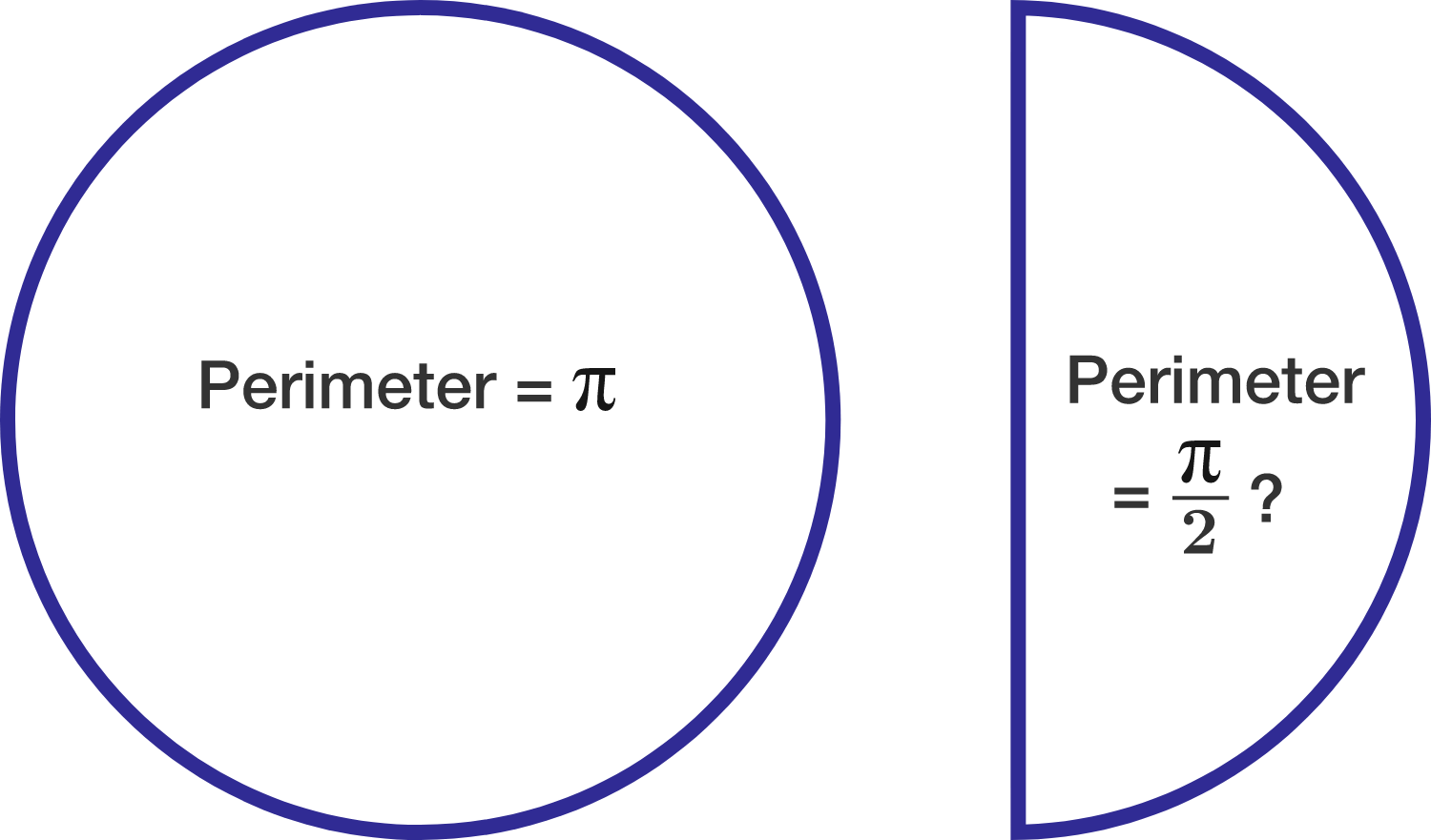# Semi: Prefix For Half

Geometry Level 2True or False?

$\quad$ If the perimeter of a circle is $\pi$, then the perimeter of a semicircle with the same radius is $\dfrac\pi2$.

×